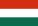matematika számolás számológép ceruzaPublished

4 Mar 2022

# Mathematical Reaction Kinetics Research GroupThe main goal of this research is the stochastic and deterministic description of the kinetics of chemical reaction networks.

#### Dr. Gábor Lente

Research group members: Rebeka Szabó

The aim of the research group is to develop mathematical methods for reaction kinetics and to refine the mathematical models of various chemical systems. In particular, the following areas are covered:

#### 1. Applications of stochastic kinetics

Stochastic kinetics, in contrast to the concept of continuous concentration in conventional reaction kinetics, considers the discrete nature of matter. Instead of specifying the time dependence of concentrations, this method describes the probability that a given number of molecules are present in a system at a selected time. Stochastic kinetics has been used successfully to interpret experimental findings in absolute chiral synthesis and also in enzyme-catalyzed systems with very low number of catalyst molecules.

#### 2. Solving reaction-diffusion equations

In experimental methods of reaction kinetics for rapid processes, it is a very common phenomenon that the homogeneity of the solution cannot be ensured due to lack of time. In such systems, it is important to use reaction-diffusion equations to interpret the experimental findings properly. The research group’s experience is related to the use of flash photolysis and stopped-flow techniques.

#### 3. Analytical solution of differential equations in reaction kinetic schemes

In multi-step reaction kinetic schemes, finding analytical solutions helps to understand how the concentration-time curves depend on the values ​​of the parameters (rate constants and initial concentrations). Recently, analytical solutions have been found for a relatively wide range of two-step processes, and also for systems containing an infinitely large number of dependent variables.